# End-to-End Bluetooth LE PHY Simulation Using Path Loss Model, RF Impairments, and AWGN

This example uses Bluetooth® Toolbox to perform end-to-end Bluetooth low energy (LE) simulation for different Bluetooth LE physical layer (PHY) transmission modes in the presence of the path loss model, radio front-end (RF) impairments, and additive white Gaussian noise (AWGN). The simulation results show the estimated value of the bit error rate (BER), path loss, and distance between the transmitter and receiver.

### Path Loss Modeling in Bluetooth LE Network

The Bluetooth Core Specifications  defined by the Bluetooth Special Interest Group (SIG) introduced Bluetooth LE to enable low-power short-range communication. Bluetooth LE devices operate in the globally unlicensed industrial, scientific, and medical (ISM) band in a frequency range from 2.4 GHz to 2.485 GHz. Bluetooth LE specifies a channel spacing of 2 MHz, resulting in 40 RF channels. The prominent applications of Bluetooth LE include direction finding services and building intelligent internet of things (IoT) solutions to facilitate home, commercial, and industrial automation. For more information about direction finding services in Bluetooth LE, see the Bluetooth Location and Direction Finding.

In past few years, there has been a significant increase in designing Bluetooth LE networks for a plethora of use case scenarios. To achieve high performance and quality in the Bluetooth LE network, studying the propagation of the Bluetooth LE signal along the link between the transmitter and the receiver is recommended. This example shows an end-to-end Bluetooth LE simulation considering these factors that impact the propagation of Bluetooth LE signals along the communication link between the transmitter and receiver.

• Environment

• Transmit power

• Antenna gain

Receiver sensitivity is the measure of minimum signal strength at which the receiver can detect, demodulate, and decode the waveform. The reference sensitivity level specified in the Bluetooth Core Specifications  is -70 dBm. However, the actual sensitivity level for the receiver as per the Bluetooth Core Specifications  is defined as the receiver input level for which the BER specified in this table is achieved.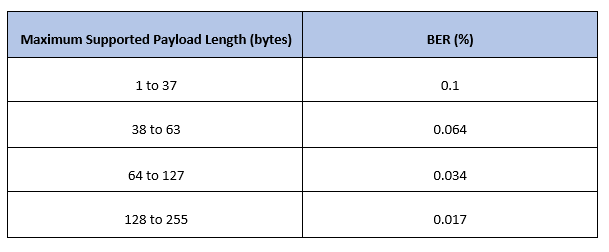This table shows the actual sensitivity level of the receiver for a given PHY transmission mode.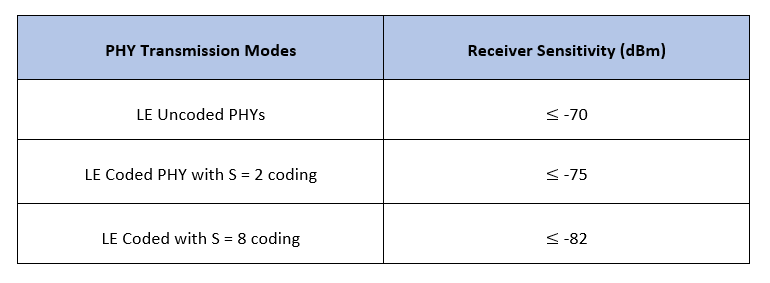#### Environment

Bluetooth LE networks are operated in different environments such as home, office, industrial, and outdoor. A specific path loss model is used for each environment.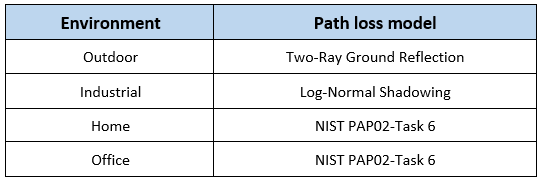#### Path Loss Model

Path loss or path attenuation is the decline in the power density of a given signal as it propagates from the transmitter to receiver through space. This reduction in power density occurs naturally over the distance and is impacted by the obstacles present in the environment in which the signal is being transmitted. The path loss is generally expressed in decibels (dB) and is calculated as:

${\mathrm{PL}}_{\mathrm{dB}}={\mathit{P}}_{\mathit{t}}-{\mathit{P}}_{\mathit{r}}$.

In this equation,

• ${\mathrm{PL}}_{\mathrm{dB}}$ is the path loss in dB.

• ${\mathit{P}}_{\mathit{t}}$ is the transmitted signal power in dB.

• ${\mathit{P}}_{\mathit{r}}$ is the received signal power in dB.

Path loss models describe the signal attenuation between the transmitter and receiver based on the propagation distance and other parameters such as frequency, wavelength, path loss exponent, and antenna gains.

#### Free-Space Path Loss Model

Free-space path loss is the attenuation of signal strength between the transmitter and receiver along the line of sight (LoS) path through free space (usually air), excluding the effect of the obstacles in the path. The free-space path loss is calculated as:

${\mathrm{PL}}_{\mathrm{dB}}=20\mathrm{log}\left(\frac{4\pi \mathit{d}}{\lambda }\right)$.

In this equation,

• $\mathit{d}$ is the distance between the transmitter and receiver.

• $\lambda$ is the signal wavelength.

#### Log-Normal Shadowing Path Loss Model

A log-distance path loss model reflects the path loss that a signal encounters in an indoor environment such as a building. The log-normal shadowing model is an extension of log-distance path loss model. Unlike the log-distance model, the log-normal shadowing model considers the fact that the surrounding environment clutter can be vastly different at two different locations having the same transmitter-receiver separation. Measurements show that at any transmitter-receiver distance, $\mathit{d}$, the path loss at a particular location is random and distributed log normally (in dB) about the mean distance dependent value. The path loss is calculated as:

${\mathrm{PL}}_{\mathrm{dB}}\left(\mathit{d}\right)={\mathrm{PL}}_{\mathrm{dB}}\left({\mathit{d}}_{0}\right)+10\gamma \mathrm{log}\left(\frac{\mathit{d}}{{\mathit{d}}_{0}}\right)+{\mathit{X}}_{\sigma }$.

In this equation,

• ${\mathrm{PL}}_{\mathrm{dB}}\left({\mathit{d}}_{0}\right)$ is the path loss at the reference distance ${\mathit{d}}_{0}$.

• $\mathit{d}$ is the distance between the transmitter and receiver.

• ${\mathit{d}}_{0}$ is the reference distance.

• $\gamma$ is the path loss exponent.

• ${\mathit{X}}_{\sigma }$ is the normal or Gaussian random variable with zero mean, reflecting the attenuation caused by the flat fading.

#### Two-Ray Ground Reflection Model

The two-ray ground reflection model  is a radio propagation model that estimates the path loss between the transmitter and receiver by considering these two signal components: LoS and the component reflected from the ground. When the transmitter and receiver antenna heights are approximately similar and the distance between the antennas is very large relative to the height of the antennas, then the path loss is calculated as:

${\mathrm{PL}}_{\mathrm{linear}\text{\hspace{0.17em}}\mathrm{scale}}=\frac{\mathit{G}\text{\hspace{0.17em}}{\mathit{h}}_{\mathit{t}}^{2}{\mathit{h}}_{\mathit{r}}^{2}}{{\mathit{d}}^{4}}$.

The path loss in logarithmic scale is calculated as:

${\mathrm{PL}}_{\mathrm{dB}}=\text{\hspace{0.17em}}40\mathrm{log10}\left(\mathit{d}\right)-10\mathrm{log10}\left(\mathit{G}\text{\hspace{0.17em}}{\mathit{h}}_{\mathit{t}}^{2}{\mathit{h}}_{\mathit{r}}^{2}\right)$.

In this equation,

• $\mathit{d}$ is the distance between the transmitter and receiver.

• $\mathit{G}$ is the product of antenna gains.

• ${\mathit{h}}_{\mathit{t}}$ is the height of the transmitter.

• ${\mathit{h}}_{\mathit{r}}$ is the height of the receiver.

The National Institute of Standards and Technology (NIST) conducted studies for indoor to indoor, outdoor to outdoor, and outdoor to indoor propagation paths and derived these equations for calculating the path loss:

`$\begin{array}{l}{\mathrm{PL}}_{\mathit{d}\text{\hspace{0.17em}}}={\mathrm{PL}}_{0}+10\left({\mathit{n}}_{0}\right)\mathrm{log10}\left(\frac{\mathit{d}}{{\mathit{d}}_{0}}\right).\text{\hspace{0.17em}\hspace{0.17em}\hspace{0.17em}\hspace{0.17em}\hspace{0.17em}\hspace{0.17em}\hspace{0.17em}\hspace{0.17em}\hspace{0.17em}\hspace{0.17em}\hspace{0.17em}\hspace{0.17em}\hspace{0.17em}\hspace{0.17em}\hspace{0.17em}\hspace{0.17em}\hspace{0.17em}\hspace{0.17em}\hspace{0.17em}\hspace{0.17em}\hspace{0.17em}\hspace{0.17em}\hspace{0.17em}\hspace{0.17em}\hspace{0.17em}\hspace{0.17em}\hspace{0.17em}\hspace{0.17em}\hspace{0.17em}\hspace{0.17em}\hspace{0.17em}\hspace{0.17em}\hspace{0.17em}\hspace{0.17em}\hspace{0.17em}\hspace{0.17em}\hspace{0.17em}\hspace{0.17em}\hspace{0.17em}\hspace{0.17em}\hspace{0.17em}\hspace{0.17em}\hspace{0.17em}\hspace{0.17em}\hspace{0.17em}\hspace{0.17em}\hspace{0.17em}\hspace{0.17em}\hspace{0.17em}\hspace{0.17em}\hspace{0.17em}\hspace{0.17em}\hspace{0.17em}\hspace{0.17em}\hspace{0.17em}\hspace{0.17em}\hspace{0.17em}\hspace{0.17em}\hspace{0.17em}}\mathrm{for}\text{\hspace{0.17em}}\mathit{d}\le {\mathit{d}}_{1}\\ {\mathrm{PL}}_{\mathit{d}\text{\hspace{0.17em}}}={\mathrm{PL}}_{0}+10\left({\mathit{n}}_{0}\right)\mathrm{log10}\left(\frac{\mathit{d}}{{\mathit{d}}_{0}}\right)+10\left({\mathit{n}}_{1}\right)\mathrm{log10}\left(\frac{\mathit{d}}{{\mathit{d}}_{1}}\right).\text{\hspace{0.17em}\hspace{0.17em}\hspace{0.17em}\hspace{0.17em}\hspace{0.17em}\hspace{0.17em}\hspace{0.17em}\hspace{0.17em}\hspace{0.17em}\hspace{0.17em}\hspace{0.17em}\hspace{0.17em}\hspace{0.17em}\hspace{0.17em}\hspace{0.17em}\hspace{0.17em}\hspace{0.17em}}\mathrm{for}\text{\hspace{0.17em}}\mathit{d}>{\mathit{d}}_{1}\end{array}$`

In these equations,

• ${\mathrm{PL}}_{0}$ is the path loss at the reference distance ${\mathit{d}}_{0}$.

• ${\mathit{n}}_{0}$,${\mathit{n}}_{1}$ are the path loss exponents.

• $\mathit{d}$ is the distance between the transmitter and receiver.

• ${\mathit{d}}_{0}$ is the reference distance, assumed to be 1 meter in simulations.

• ${\mathit{d}}_{1}$ is the breakpoint where the path loss exponent adjusts from ${\mathit{n}}_{0}$ to ${\mathit{n}}_{1}$.

The example considers these values for different environments.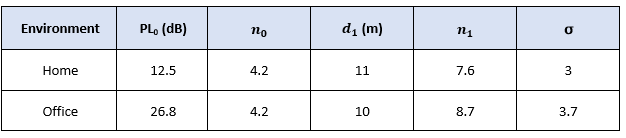Most of these measurements for the NIST PAP02 Task 6 channel model were taken with transmitters and receivers located in hallways with distances ranging from 5 m to 45 m.

#### Transmit Power

Transmit power is the power of the radio frequency signal generated by the transmitter. Increasing the transmit power increases the likelihood that the signal can be transmitted over longer distances. Bluetooth supports transmit power from -20 dBm (0.01 mW) to 20 dBm (100 mW).

#### Antenna Gain

Antenna gain is the factor by which the antenna improves the total radiated power. Bluetooth designers can choose to implement a variety of antenna options. Bluetooth devices typically achieve an antenna gain in the range from -10 dBi to 10 dBi.

### End-to-End Bluetooth LE Simulation Procedure

The end-to-end Bluetooth LE PHY simulations estimate the BER and the distance between the transmitter and receiver by considering a specific environment with RF impairments and AWGN added to the transmission packets.

For a given set of simulation parameters, obtain the signal-to-noise ratio (SNR) at the receiver by assuming a fixed noise figure. For the obtained value of SNR including the path loss, generate the Bluetooth LE waveform using `bleWaveformGenerator` function. Distort the generated waveform with RF impairments and AWGN. Each packet is distorted by these RF impairments:

• DC offset

• Carrier frequency offset

• Carrier phase offset

• Timing drift

The noisy packets are processed through a practical Bluetooth LE receiver that performs these operations:

1. Automatic gain control (AGC)

2. DC removal

3. Carrier frequency offset correction

4. Matched filtering

5. Packet detection

6. Timing error detection

7. Demodulation and decoding

8. De-whitening

The end-to-end example chain is summarized in these block diagrams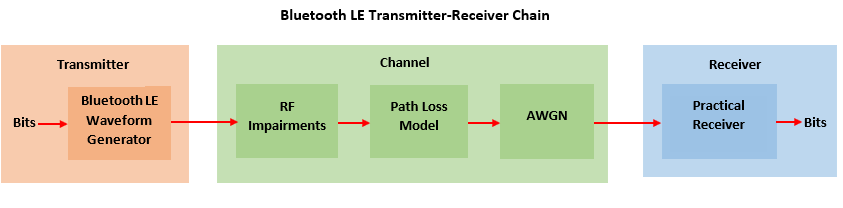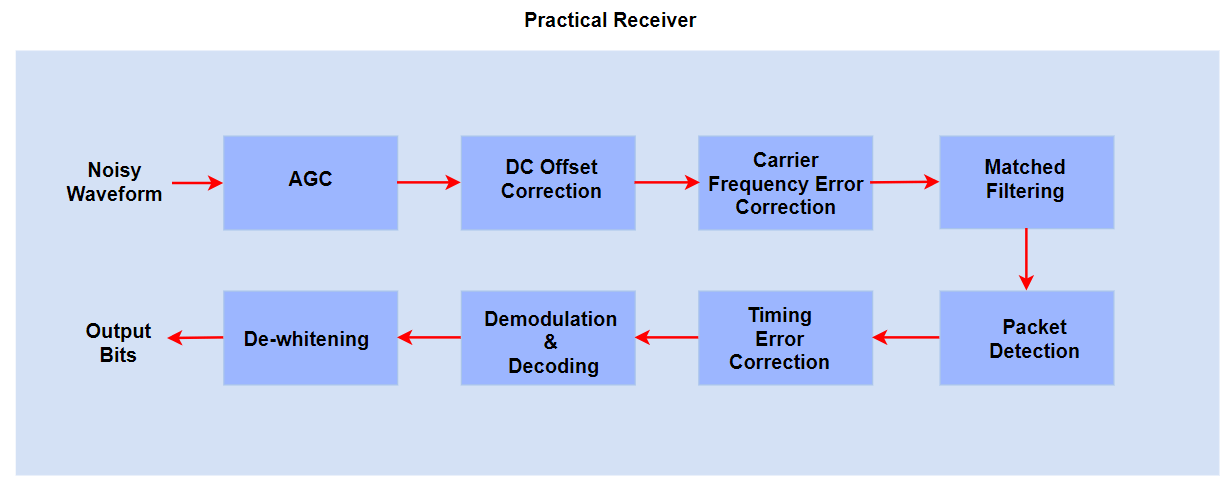The BER is obtained by comparing the transmitted and recovered data bits.

### Configure Simulation Parameters

In this example, the distance between the transmitter and receiver is estimated based on the environment and the power levels of the signal at the transmitter and receiver. Configure the parameters using the `bluetoothRangeConfig` object.

#### Configure parameters related to the communication link between the transmitter and receiver

```rangeConfig = bluetoothRangeConfig; rangeConfig.Environment =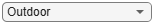"Outdoor"; % Environment rangeConfig.Mode =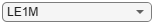'LE1M'; % PHY transmission mode rangeConfig.ReceiverSensitivity =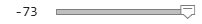-73 ; % Receiver sensitivity in dBm rangeConfig.TransmitterPower =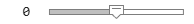0; % Transmit power in dBm rangeConfig.TransmitterAntennaGain =0; % Transmitter antenna gain in dB rangeConfig.ReceiverAntennaGain =0; % Receiver antenna gain in dB if strcmp(rangeConfig.Environment,'Industrial') % Link margin(dB) assumed in the simulation rangeConfig.LinkMargin = 7 elseif strcmp(rangeConfig.Environment,'Outdoor') rangeConfig.LinkMargin = 15 else rangeConfig.LinkMargin = 0 end ```
```rangeConfig = bluetoothRangeConfig with properties: Environment: 'Outdoor' SignalPowerType: 'ReceiverSensitivity' Mode: 'LE1M' ReceiverSensitivity: -73 LinkMargin: 15 TransmitterPower: 0 TransmitterAntennaGain: 0 ReceiverAntennaGain: 0 TransmitterCableLoss: 1.2500 ReceiverCableLoss: 1.2500 TransmitterAntennaHeight: 1 ReceiverAntennaHeight: 1 Read-only properties: FSPLDistance: 5.8256 PathLossModel: 'TwoRayGroundReflection' ```

#### Configure parameters for waveform generation

```sps = 8; % Samples per symbol dataLen =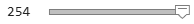254; % Data length in bytes channelIndex =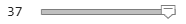37; % Random channel index```

#### Configure RF impairments

```frequencyOffset =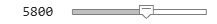5800; % Frequency offset in Hz phaseOffset =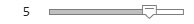5; % Phase offset in degrees initoff = 0.15*sps; % Static timing offset stepsize = 20*1e-6; % Timing drift in ppm, Max range is +/- 50 ppm dcOffset = 20; % Percentage related to maximum amplitude value```

### Generate Bluetooth LE Waveform

Generate Bluetooth LE waveform based on waveform configuration parameters.

```% Default access address for periodic advertising channels accessAddress = [0 1 1 0 1 0 1 1 0 1 1 1 1 1 0 1 1 0 0 1 0 0 0 1 0 1 1 1 0 0 0 1]'; % Random data bits generation txBits = randi([0 1],dataLen*8,1,'int8'); % Generate Bluetooth LE waveform txWaveform = bleWaveformGenerator(txBits,'Mode',rangeConfig.Mode,... 'SamplesPerSymbol',sps,... 'ChannelIndex',channelIndex,... 'AccessAddress',accessAddress);```

#### Configure noise and signal power at the receiver

The noise floor of the receiver is simulated with thermal noise. The height of the noise floor determines the SNR at the receiver. The noise figure of the receiver determines the level of noise floor.

```NF = 6; % Noise figure (dB) T = 290; % Ambient temperature (K) dBm2dBFactor = 30; % Factor for converting dBm to dB % Symbol rate based on the PHY transmission mode symbolRate = 1e6; if strcmp(rangeConfig.Mode,'LE2M') symbolRate = 2e6; end BW = sps*symbolRate; % Bandwidth (Hz) k = 1.3806e-23; % Boltzmann constant (J/K) noiseFloor = 10*log10(k*T*BW)+NF; % Nosie floor in dB % Measure the path loss and signal power at the receiver. [pldB,sigPowerdBm] = pathLoss(rangeConfig); measuredPowerVector = sigPowerdBm - dBm2dBFactor; snrdB = measuredPowerVector - noiseFloor; % SNR in dB plLinear = 10^(pldB/20); % Convert path loss from dB to linear scale```

### Distort Bluetooth LE Waveform

Distort the generated Bluetooth LE waveform using RF impairments, path loss, and AWGN.

The RF impairments are generated randomly and added to the Bluetooth LE waveform.

```% Create and configure the System objects for impairments initImp = helperBLEImpairmentsInit(rangeConfig.Mode,sps); % Configure RF impairments initImp.pfo.FrequencyOffset = frequencyOffset; % Frequency offset in Hz initImp.pfo.PhaseOffset = phaseOffset; % Phase offset in degrees initImp.vdelay = (initoff:stepsize:initoff+stepsize*(length(txWaveform)-1))'; initImp.dc = dcOffset; % Pass generated Bluetooth LE waveform through RF impairments txImpairedWfm = helperBLEImpairmentsAddition(txWaveform,initImp);```

#### Attenuate Impaired Bluetooth LE Waveform

Attenuate the impaired Bluetooth LE waveform.

```% Attenuate Bluetooth LE waveform attenWaveform = txImpairedWfm.*10^(measuredPowerVector/20);```

Add AWGN to the attenuated Bluetooth LE waveform.

`rxWaveform = awgn(attenWaveform,snrdB,'measured');`

### Simulation Results

Estimate and display the BER and the distance between the transmitter and the receiver by processing the distorted Bluetooth LE waveform through the practical receiver.

To retrieve the data bits, pass the attenuated, AWGN-distorted Bluetooth LE waveform through the practical receiver.

```% Configure the receiver parameters in a structure rxCfg = struct(Mode=rangeConfig.Mode,SamplesPerSymbol=sps,ChannelIndex=channelIndex,... DFPacketType='Disabled',AccessAddress=accessAddress); rxCfg.CoarseFreqCompensator = comm.CoarseFrequencyCompensator(Modulation="OQPSK",... SampleRate=BW,... SamplesPerSymbol=2*sps,... FrequencyResolution=100); rxCfg.PreambleDetector = comm.PreambleDetector(Detections="First"); % Recover data bits using practical receiver [rxBits,accessAddress] = helperBLEPracticalReceiver(rxWaveform,rxCfg);```

#### Estimate BER

Estimate value of the BER based on the retrieved and the transmitted data bits.

```% Obtain BER by comparing the transmitted and recovered bits if(length(txBits) == length(rxBits)) ber = (sum(xor(txBits,rxBits))/length(txBits)); else disp('Unable to compute BER due to length mismatch in input and decoded bits') end```

#### Estimate Distance

Estimate the distance between the transmitter and the receiver.

```% Estimate the distance between the transmitter and the receiver based on the environment distance = bluetoothRange(rangeConfig);```

#### Display Results

Display the estimated results and plot the spectrum of the transmitted and received Bluetooth LE waveform.

```% Display estimated BER and distance between the transmitter and the receiver. disp(['Input configuration: ', newline , ' PHY transmission mode: ', rangeConfig.Mode,.... newline,' Environment: ', rangeConfig.Environment]);```
```Input configuration: PHY transmission mode: LE1M Environment: Outdoor ```
```disp(['Estimated outputs: ', newline , ' Path loss : ', num2str(pldB), ' dB'.... newline,' Distance between the transmitter and receiver: ', num2str(round(distance(1))), ' to ', num2str(round(distance(2))), ' m', newline, ... ' Free space path loss distance: ', num2str(round(rangeConfig.FSPLDistance)), ' m', newline, ' BER: ', num2str(ber)]);```
```Estimated outputs: Path loss : 55.5 dB Distance between the transmitter and receiver: 5 to 8 m Free space path loss distance: 6 m BER: 0 ```
```% Plot the spectrum of the transmitted and received Bluetooth LE waveform specAnalyzer = dsp.SpectrumAnalyzer('NumInputPorts',2,'SampleRate',symbolRate*sps,... 'Title','Spectrum of Transmitted and Received Bluetooth LE Signals',... 'ShowLegend',true,'ChannelNames',{'Transmitted Bluetooth LE signal','Received Bluetooth LE signal'}); specAnalyzer(txWaveform,rxWaveform); release(specAnalyzer);```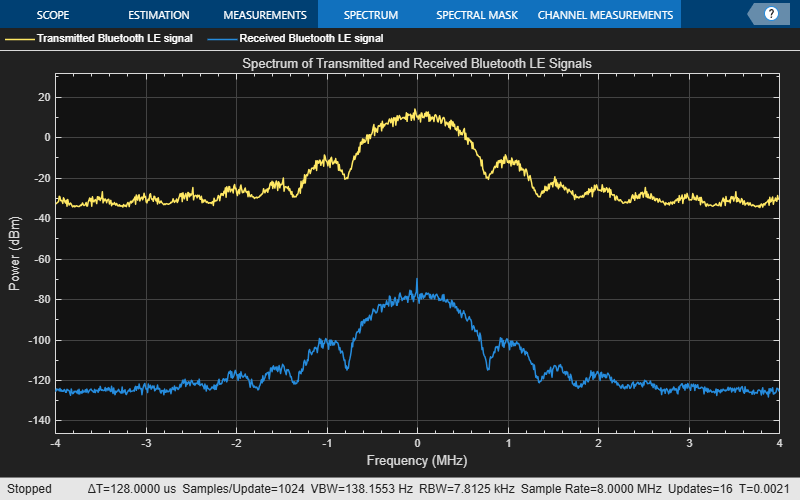This example demonstrates an end-to-end Bluetooth LE simulation for different PHY transmission modes by considering the path loss model, RF impairments, and AWGN. The obtained simulation results display the path loss, estimated distance between the transmitter and receiver, and BER. The spectrum of the transmitted and received Bluetooth LE waveform is visualized by using a spectrum analyzer.

### Appendix

The example uses these helper functions:

### Selected Bibliography

 Bluetooth Special Interest Group (SIG). "Bluetooth Core Specification". Version 5.3. https://www.bluetooth.com.

 Path Loss Models Used in Bluetooth Range Estimator. Bluetooth Special Interest Group (SIG). https://www.bluetooth.com.

 Rappaport, Theodore. Wireless Communication – Principles and Practice. Prentice Hall, 1996.

 NIST Smart Grid Interoperability Panel Priority Action Plan 2: Guidelines for Assessing Wireless Standards for Smart Grid Applications. National Institute of Standards and Technology, U.S. Department of Commerce, 2014, https://nvlpubs.nist.gov/.